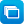Search results

Year level
Resource type
Learning area

Refine by topic

Main topic Specific topic Related topic
Listed under:  GeometryTIMES Module 26: Measurement and Geometry: circle geometry - teacher guide

This is a 44-page guide for teachers. It contains theorems on chords, arcs, angles and secants associated with circles.Using 'Cities taking shape' - Teacher idea

This Teacher idea includes comments following the teaching of R11361 'Cities taking shape - unit of work' to a years 4-5 class. The unit promotes students' knowledge of 2D and 3D shapes, and the relationship between them. It offers interactive and hands-on tasks to develop, consolidate and extend students' understandings ...TIMES Module 13: Measurement and Geometry: construction - teacher guide

This is a 30-page guide for teachers that explains the central role of construction and presents examples of constructions.TIMES Module 21: Measurement and Geometry: rhombuses, kites and trapezia - teacher guide

This is a 32-page guide for teachers. It contains the definitions, properties and tests for rhombuses, kites and trapezia. Proofs of the properties and tests are given. Constructions for rhombuses, kites and trapezia are provided.Starting Smart: how can I use measurement relationships to solve problems? - years 7-9

This curriculum resource package is a ten-week middle years teaching plan and set of supporting resources to extend students' knowledge and skills in the measurement of area and volume and to develop their ability to solve practical measurement problems. Students use counting methods and develop formulas to calculate areas ...Measuring our coastline

How long is the Australian coastline? See Dr Derek Muller and Simon Pampena discussing the perimeter of the Australian coastline. Find out how the accuracy of that measurement depends on the length of the 'measuring stick' used. They discuss how a coastline is much like a fractal such as 'Koch's Snowflake'!Visualising two-dimensional and three-dimensional shapes

This collection comprises 24 digital curriculum resources, including learning objects from the series 'Shape sorter', 'Face painter', 'Geoboard', 'Photo hunt', 'Viewfinder' and 'Shape maker'. There are three categories: exploring two-dimensional shapes; visualising three-dimensional shapes; and making three-dimensional ...TIMES Module 11: Measurement and Geometry: area, volume and surface area - teacher guide

This is a 15-page guide for teachers containing explanations of the derivation of formulas for the areas of parallelograms, trapeziums, rhombuses and kites. Formulas for the volumes and surface areas of prisms and cylinders are obtained. Applications of these formulas are given. A history of the development of these concepts ...TIMES Module 20: Measurement and Geometry: parallelograms and rectangles - teacher guide

This is a 17-page guide for teachers. It contains the definitions, properties and tests for parallelograms and rectangles. Proofs of the properties and tests are given. Constructions for parallelograms and rectangles are given.Starting Smart: how are shapes and objects related?

This curriculum resource package is a ten-week middle years teaching plan and set of supporting resources to extend students' understanding of geometrical language and spatial relationships. Students use concrete materials and interactive geometry software packages to compare and describe geometrical attributes and investigate ...Delivering water

This is a mathematics unit of work about water: its world-wide availability and use; the time spent carrying it; the best shape for water tanks; and the area of land taken up around tanks and in paths and ditches to water sources. Intended for years 9 and 10 and written from a global education perspective, the resource ...Secondary mathematics: different representations

These seven learning activities, which focus on 'representations' using a variety of tools (software) and devices (hardware), illustrate the ways in which content, pedagogy and technology can be successfully and effectively integrated in order to promote learning. In the activities, teachers use different representations ...Measures: scaling down

Compare the areas of squares, rectangles and triangles before and after being scaled down (reduced). Notice that 'similar shapes' in the mathematical sense have the same shape but different areas. Explore the relationship between side-length reduction and area reduction when scaling down shapes. This learning object is ...Measures: scaling up solids

Compare the volumes of cubes and rectangular prisms before and after being scaled up (enlarged). Notice that 'similar solids' in the mathematical sense have the same shape but different volumes. Explore the relationship between side length and volume when scaling up solids. This learning object is the fifth in a series ...Measures: volumes

Compare the volumes of a range of rectangular prisms when scaling up (enlarging) side lengths by different ratios. Notice that the rectangular prisms are not similar in the mathematical sense, but it is possible to predict the effect on the volume produced by the scaling of the sides. Identify and describe the relationship ...Congruent triangles

Find out about congruent and non-congruent triangles and the conditions required to make them. Use line segments and angles to build two congruent triangles for three different combinations of sides and angles. Explore the SSS case (side, side, side), the SAS case (two sides and the included angle) and the ASA case (two ...Exploring relationships of angles

Explore angles formed by a transversal line intersecting parallel lines. Look at illustrations showing pairs of angles: vertically opposite, corresponding and alternate angles. Name pairs of angles to score points and help a monkey drive to the supermarket to buy food.TIMES Module 22: Measurement and Geometry: introductory trigonometry - teacher guide

This is a 20-page guide for teachers containing an introduction to the three basic trigonometric ratios. A history of trigonometry concludes the module.TIMES Module 10: Measurement and Geometry: introduction to measurement - teacher guide

This is a 16-page guide for teachers. It provides an introduction to the initial ideas of measurement, and introduces the measurement of length, area, volume and time.TIMES Module 9: Measurement and Geometry: introduction to plane geometry - teacher guide

This is a 16-page guide for teachers. It provides an introduction to the initial ideas of plane geometry. Points and lines are introduced as fundamental objects in the study of geometry. Angles and parallelism are the initial areas of attention in a more formal approach to geometry that occurs from year 7.